# DERIVATIVE OF A FUNCTION is called the derivative

• Slides: 77DERIVATIVE OF A FUNCTION is called the derivative of We write: “The derivative of f with respect to x is …” There are many ways to write the derivative of at .DERIVATIVE OF A FUNCTION “f dash x” or “the derivative of f with respect to x” “y prime or dash” “dee why dee ecks” or “the derivative of y with respect to x” “dee eff dee ecks” or “the derivative of f with respect to x” “dee ecks uv eff uv ecks” or “the derivative of f of x”DERIVATIVE OF A FUNCTION dx does not mean d times x ! dy does not mean d times y !DERIVATIVE OF A FUNCTION does not mean ! (except when it is convenient to think of it as division. )DERIVATIVE OF A FUNCTION does not mean times ! (except when it is convenient to treat it that way. )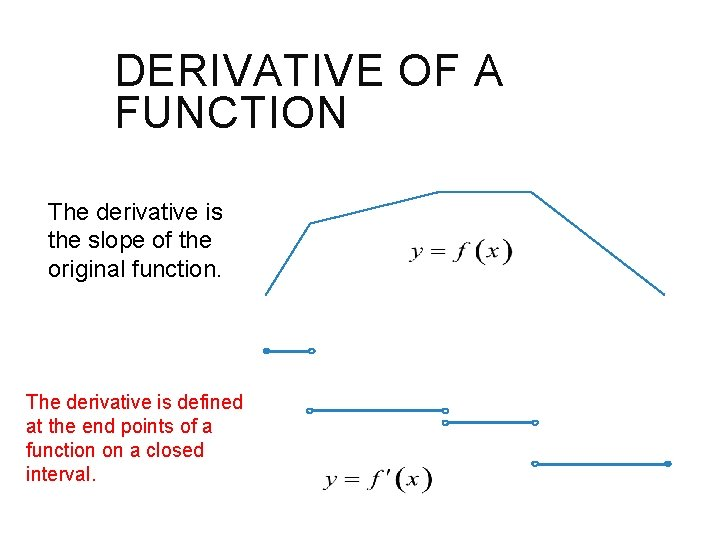DERIVATIVE OF A FUNCTION The derivative is the slope of the original function. The derivative is defined at the end points of a function on a closed interval.DERIVATIVE OF A FUNCTION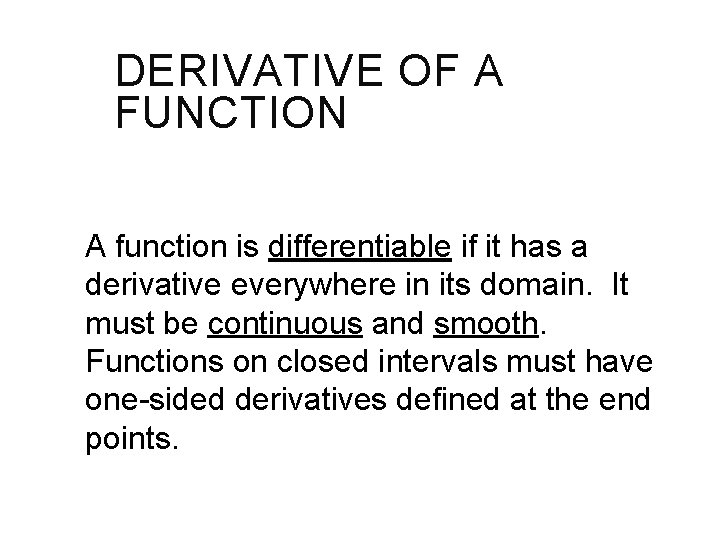DERIVATIVE OF A FUNCTION A function is differentiable if it has a derivative everywhere in its domain. It must be continuous and smooth. Functions on closed intervals must have one-sided derivatives defined at the end points.DIFFERENTIABILITY To be differentiable, a function must be continuous and smooth. Derivatives will fail to exist at: corner cusp vertical tangent discontinuity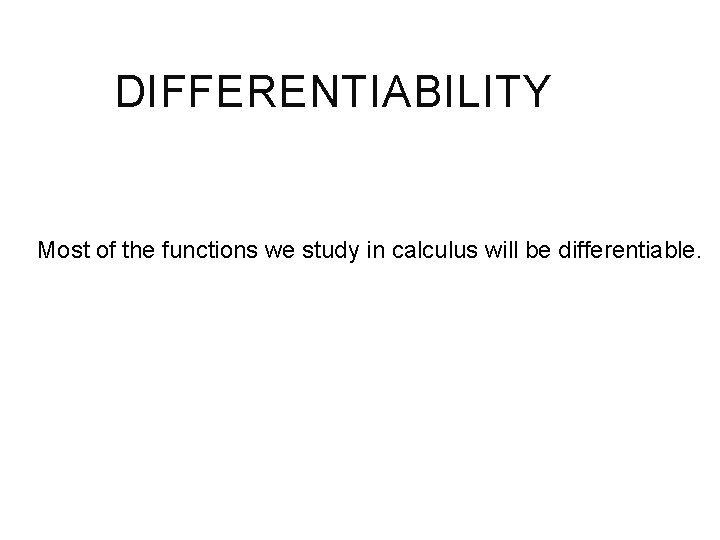DIFFERENTIABILITY Most of the functions we study in calculus will be differentiable.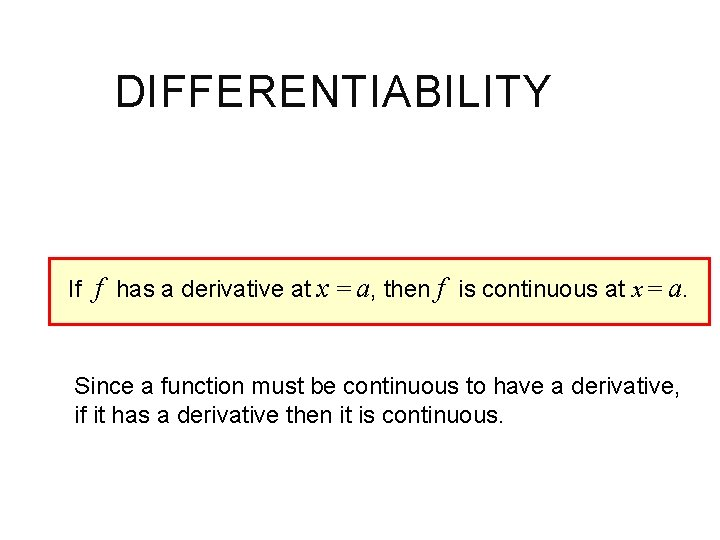DIFFERENTIABILITY If f has a derivative at x = a, then f is continuous at x = a. Since a function must be continuous to have a derivative, if it has a derivative then it is continuous.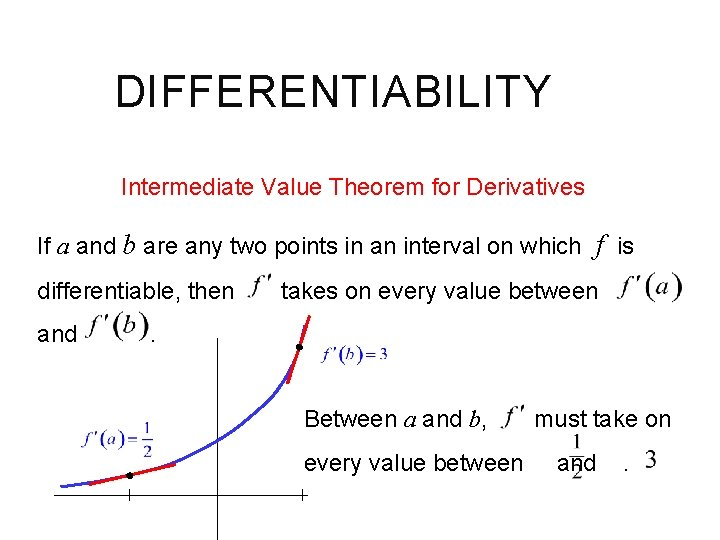DIFFERENTIABILITY Intermediate Value Theorem for Derivatives If a and b are any two points in an interval on which f is differentiable, then and takes on every value between . Between a and b, every value between must take on and .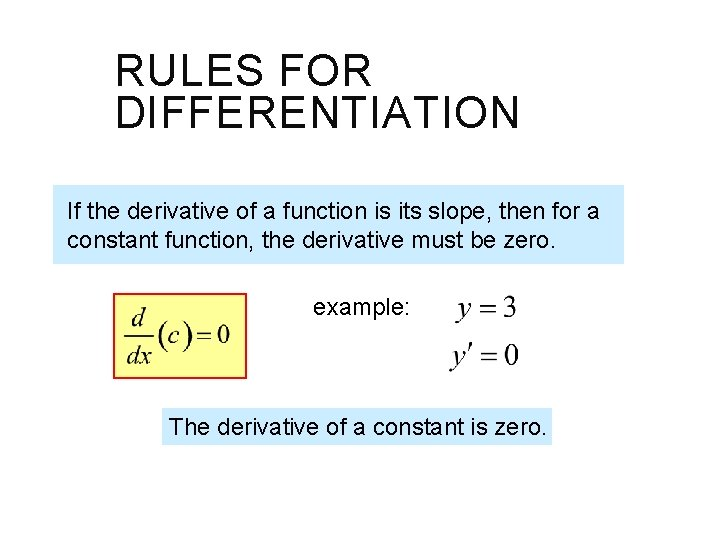RULES FOR DIFFERENTIATION If the derivative of a function is its slope, then for a constant function, the derivative must be zero. example: The derivative of a constant is zero.RULES FOR DIFFERENTIATION We saw that if , This is part of a pattern. examples: power rule .RULES FOR DIFFERENTIATION Proof: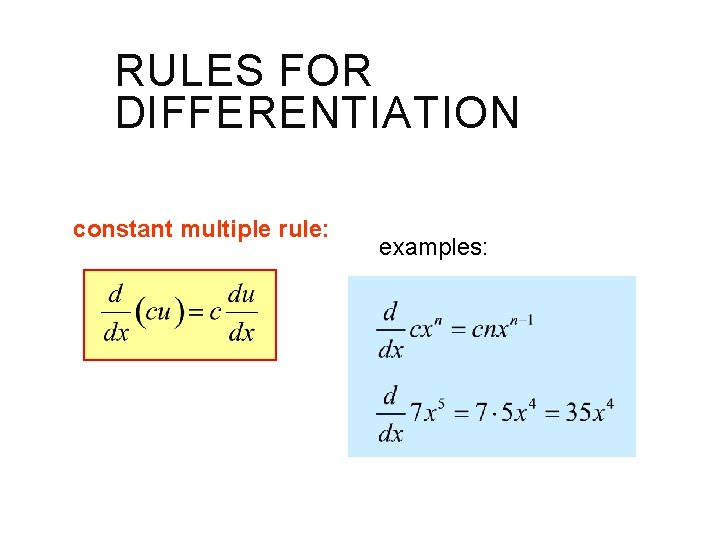RULES FOR DIFFERENTIATION constant multiple rule: examples:RULES FOR DIFFERENTIATION constant multiple rule: sum and difference rules: (Each term is treated separately)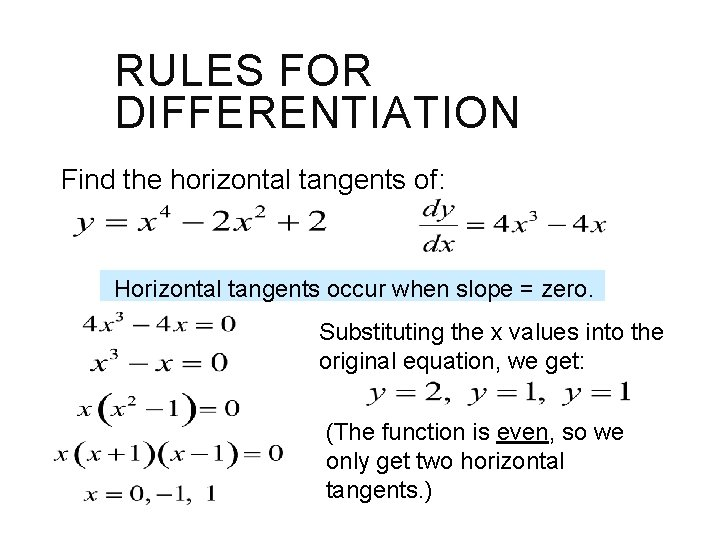RULES FOR DIFFERENTIATION Find the horizontal tangents of: Horizontal tangents occur when slope = zero. Substituting the x values into the original equation, we get: (The function is even, so we only get two horizontal tangents. )RULES FOR DIFFERENTIATION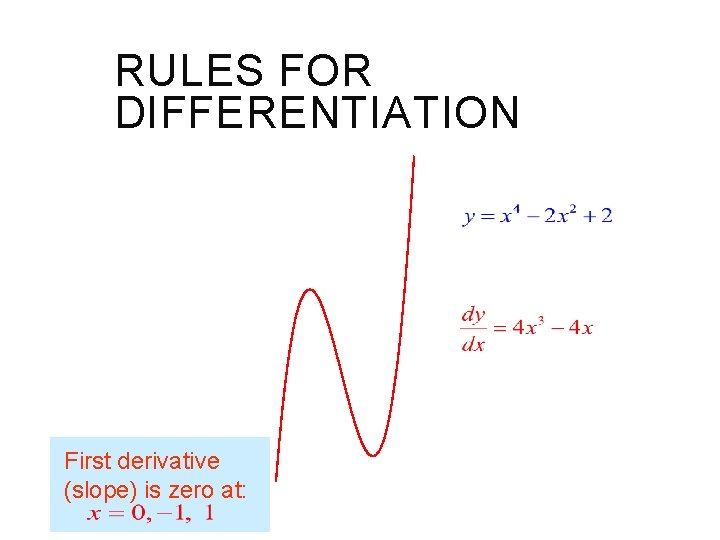RULES FOR DIFFERENTIATION First derivative (slope) is zero at: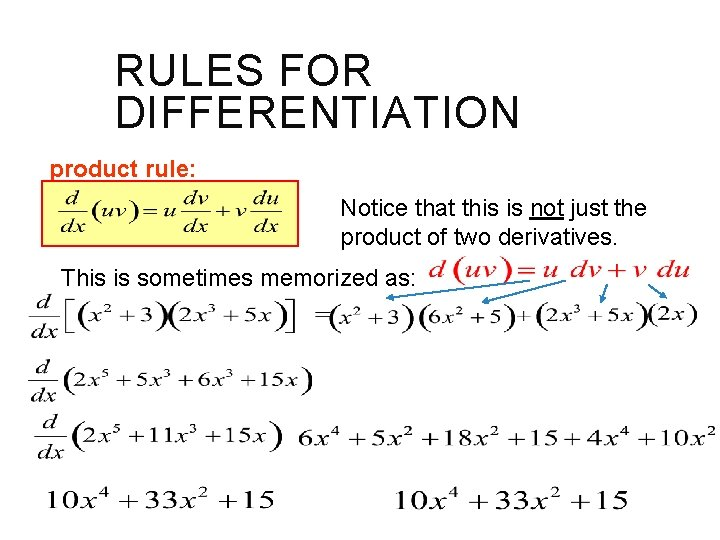RULES FOR DIFFERENTIATION product rule: Notice that this is not just the product of two derivatives. This is sometimes memorized as: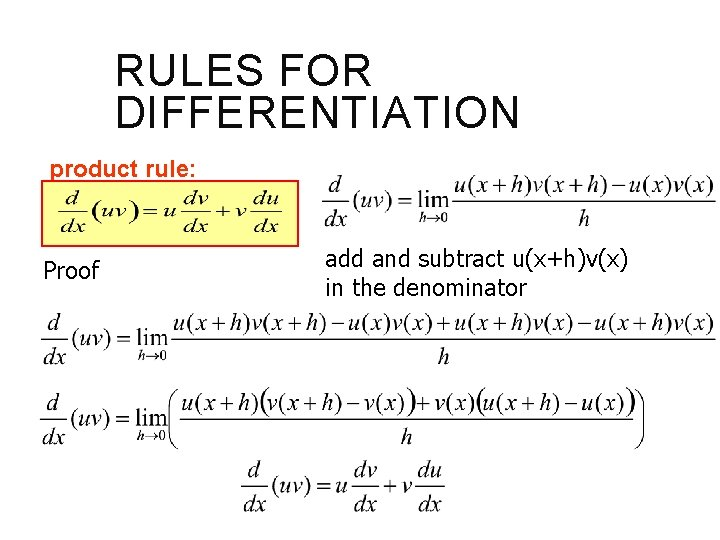RULES FOR DIFFERENTIATION product rule: Proof add and subtract u(x+h)v(x) in the denominator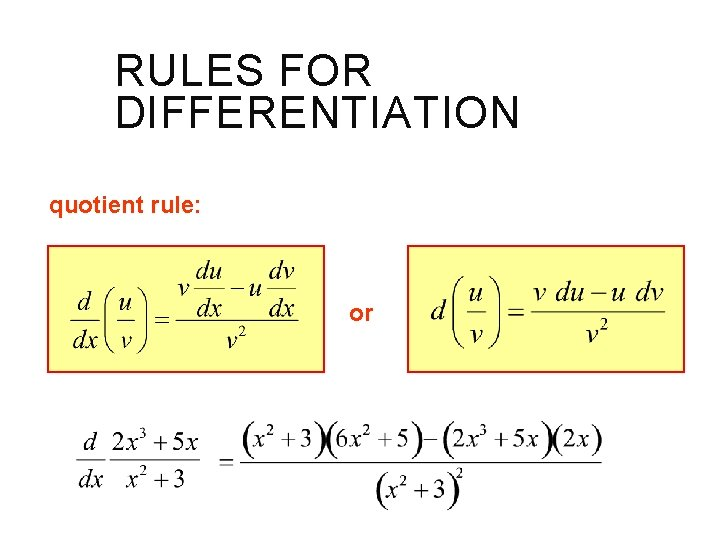RULES FOR DIFFERENTIATION quotient rule: orRULES FOR DIFFERENTIATION Higher Order Derivatives: is the first derivative of y with respect to x. is the second derivative. (y double prime) is the third derivative. is the fourth derivative. We will learn later what these higher order derivatives are used for.RULES FOR DIFFERENTIATION Suppose u and v are functions that are differentiable at x = 3, and that u(3) = 5, u’(3) = -7, v(3) = 1, and v’(3)= 4. Find the following at x = 3 :RULES FOR DIFFERENTIATIONRULES FOR DIFFERENTIATIONVELOCITY AND OTHER RATES OF CHANGE Consider a graph of displacement (distance traveled) vs. time. distance (miles) B Average velocity can be found by taking: A time (hours) The speedometer in your car does not measure average velocity, but instantaneous velocity. (The velocity at one moment in time. )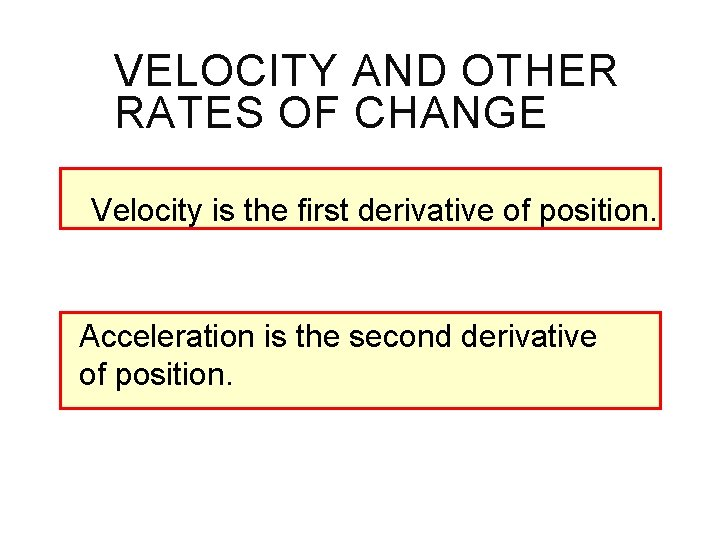VELOCITY AND OTHER RATES OF CHANGE Velocity is the first derivative of position. Acceleration is the second derivative of position.VELOCITY AND OTHER RATES OF CHANGE Example: Free Fall Equation Speed is the absolute value of velocity. Gravitational Constants:VELOCITY AND OTHER RATES OF CHANGE Acceleration is the derivative of velocity. example: If distance is in: Velocity would be in: Acceleration would be in: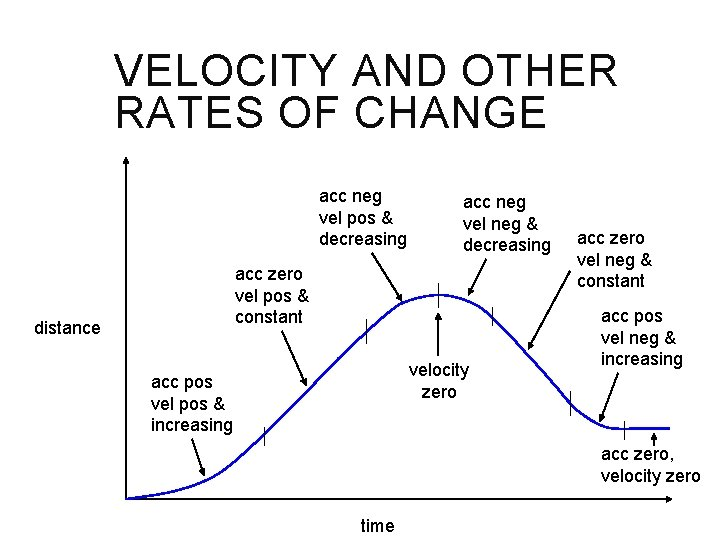VELOCITY AND OTHER RATES OF CHANGE acc neg vel pos & decreasing acc neg vel neg & decreasing acc zero vel pos & constant distance velocity zero acc pos vel pos & increasing acc zero vel neg & constant acc pos vel neg & increasing acc zero, velocity zero timeVELOCITY AND OTHER RATES OF CHANGE Rates of Change: Average rate of change = Instantaneous rate of change = These definitions are true for any function. ( x does not have to represent time. )VELOCITY AND OTHER RATES OF CHANGE For a circle: Instantaneous rate of change of the area with respect to the radius. For tree ring growth, if the change in area is constant then dr must get smaller as r gets larger.VELOCITY AND OTHER RATES OF CHANGE from Economics: Marginal cost is the first derivative of the cost function, and represents an approximation of the cost of producing one more unit.VELOCITY AND OTHER RATES OF CHANGE Example 13: Suppose it costs: to produce x stoves. If you are currently producing 10 stoves, the 11 th stove will cost approximately: The actual cost is: marginal cost actual costVELOCITY AND OTHER RATES OF CHANGE Note that this is not a great approximation – Don’t let that bother you. Marginal cost is a linear approximation of a curved function. For large values it gives a good approximation of the cost of producing the next item.VELOCITY AND OTHER RATES OF CHANGE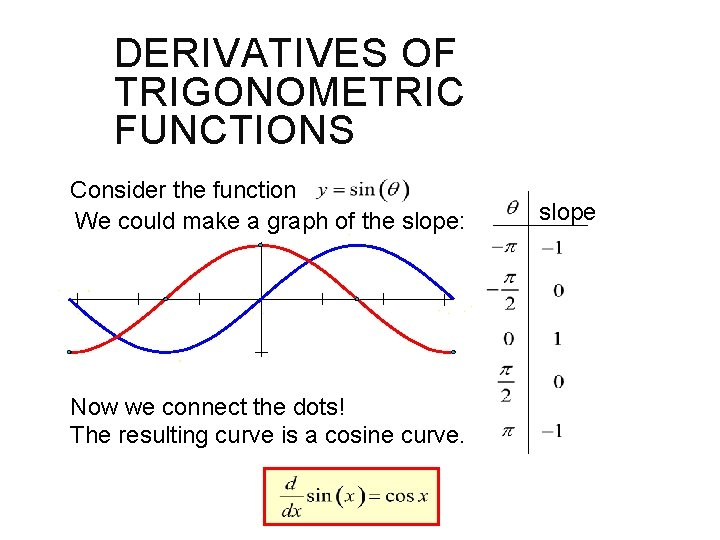DERIVATIVES OF TRIGONOMETRIC FUNCTIONS Consider the function We could make a graph of the slope: Now we connect the dots! The resulting curve is a cosine curve. slope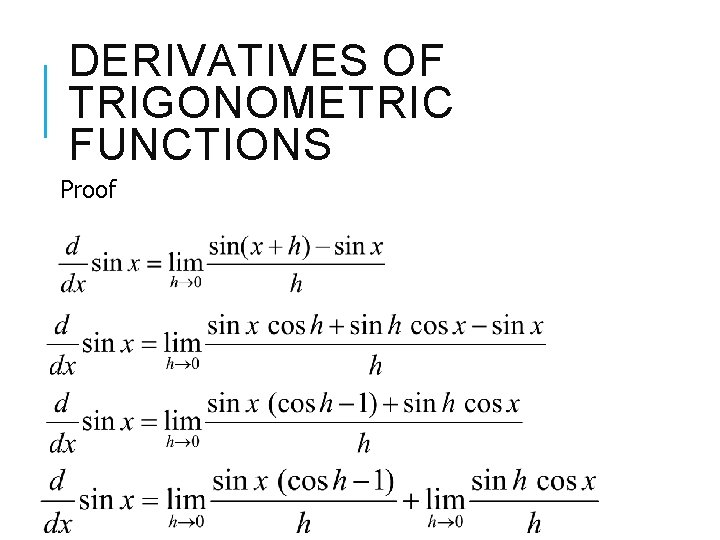DERIVATIVES OF TRIGONOMETRIC FUNCTIONS Proof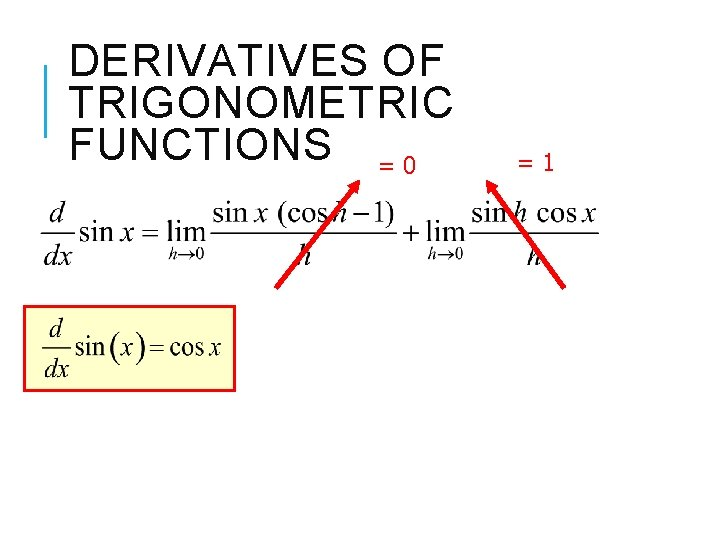DERIVATIVES OF TRIGONOMETRIC FUNCTIONS = 0 =1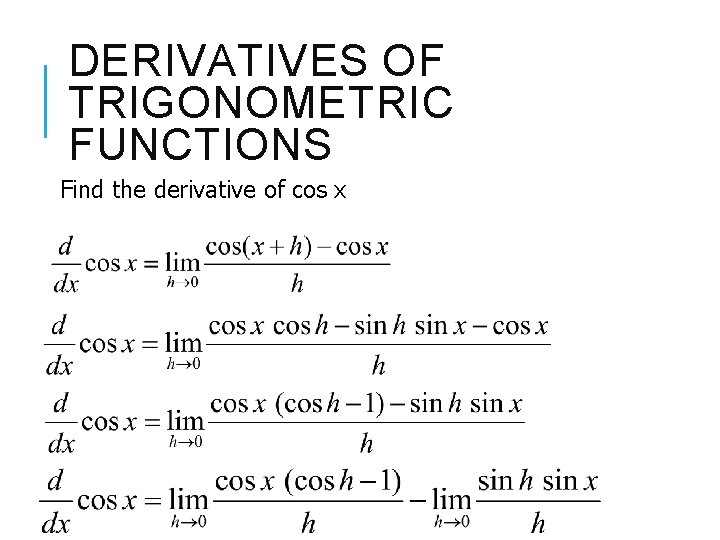DERIVATIVES OF TRIGONOMETRIC FUNCTIONS Find the derivative of cos xDERIVATIVES OF TRIGONOMETRIC FUNCTIONS = 0 =1DERIVATIVES OF TRIGONOMETRIC FUNCTIONS We can find the derivative of tangent x by using the quotient rule.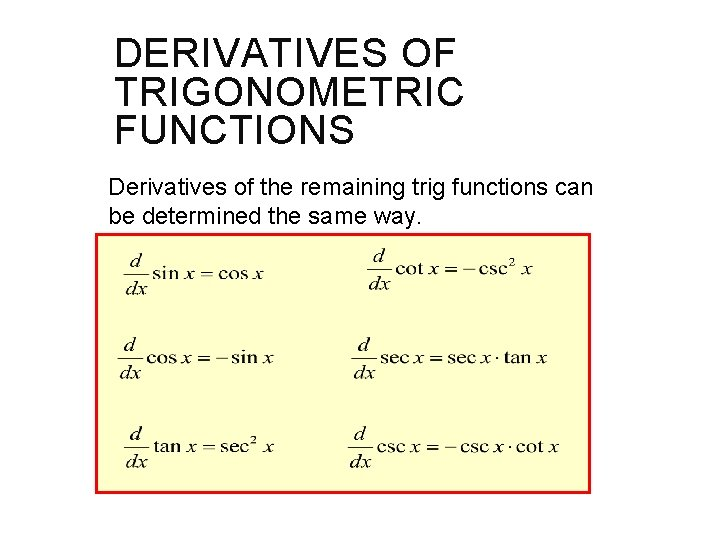DERIVATIVES OF TRIGONOMETRIC FUNCTIONS Derivatives of the remaining trig functions can be determined the same way.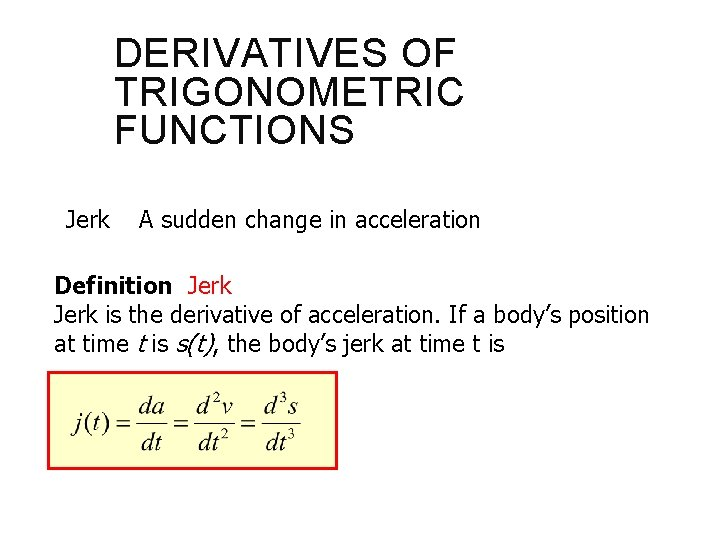DERIVATIVES OF TRIGONOMETRIC FUNCTIONS Jerk A sudden change in acceleration Definition Jerk is the derivative of acceleration. If a body’s position at time t is s(t), the body’s jerk at time t is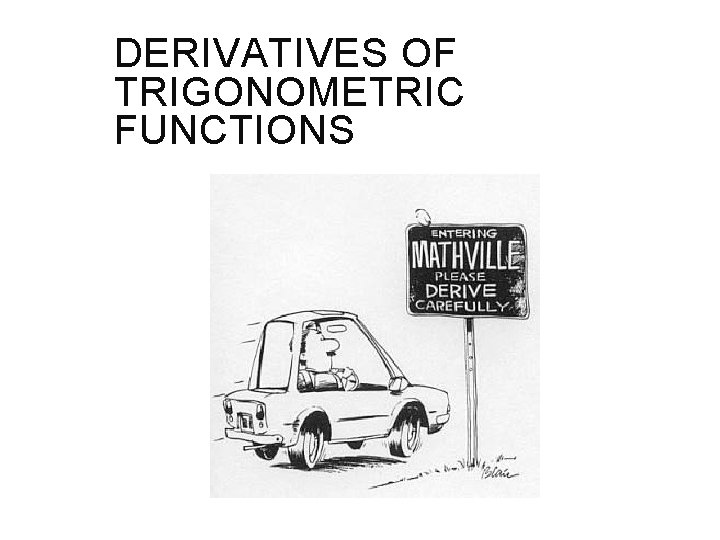DERIVATIVES OF TRIGONOMETRIC FUNCTIONS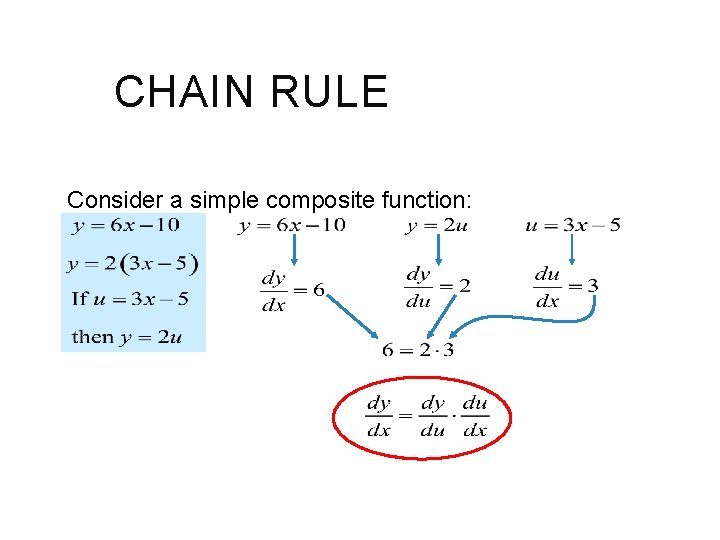CHAIN RULE Consider a simple composite function: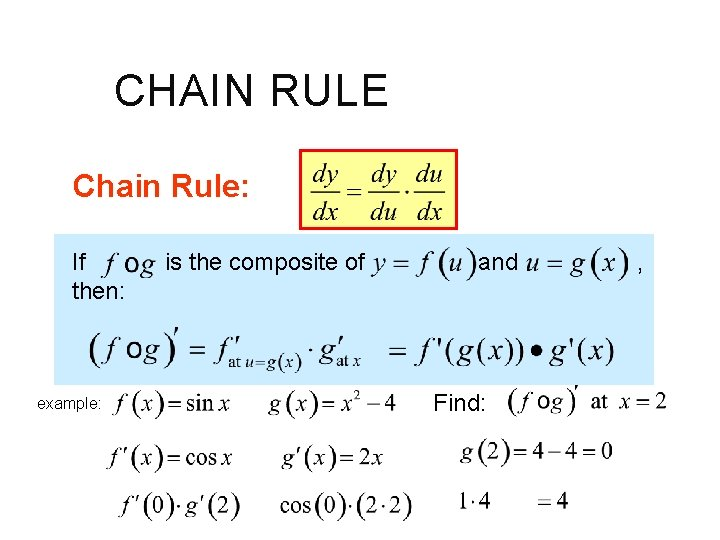CHAIN RULE Chain Rule: If then: example: is the composite of and Find: ,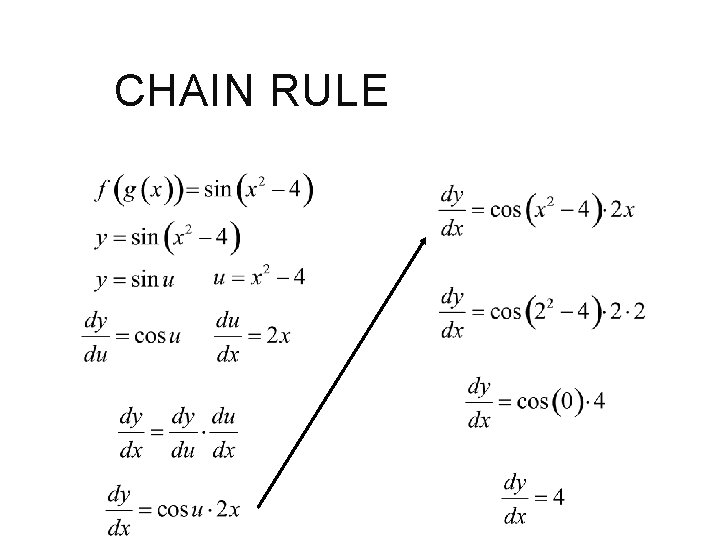CHAIN RULE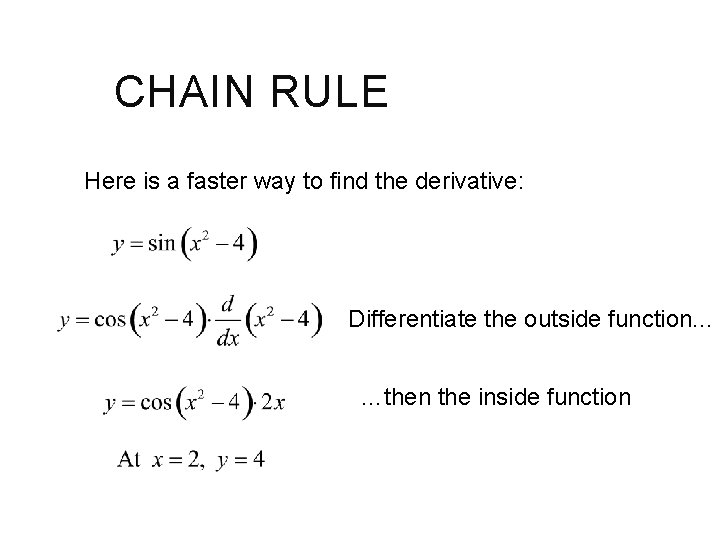CHAIN RULE Here is a faster way to find the derivative: Differentiate the outside function. . . …then the inside functionCHAIN RULE The chain rule can be used more than once. (That’s what makes the “chain” in the “chain rule”!)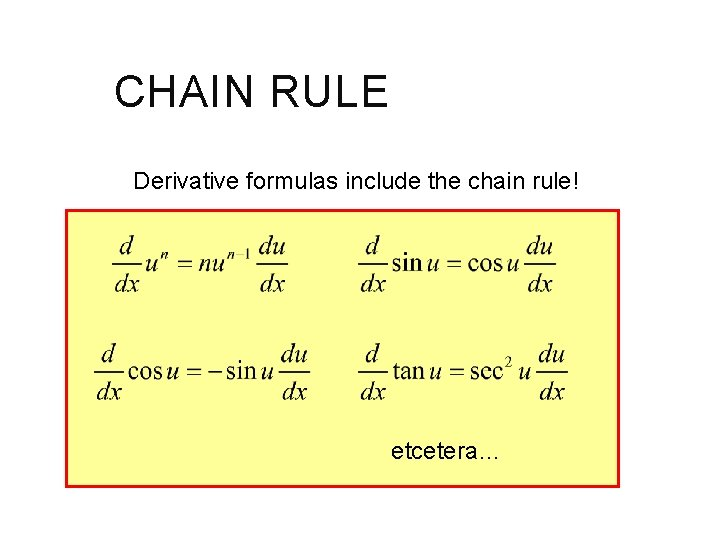CHAIN RULE Derivative formulas include the chain rule! etcetera…CHAIN RULE Find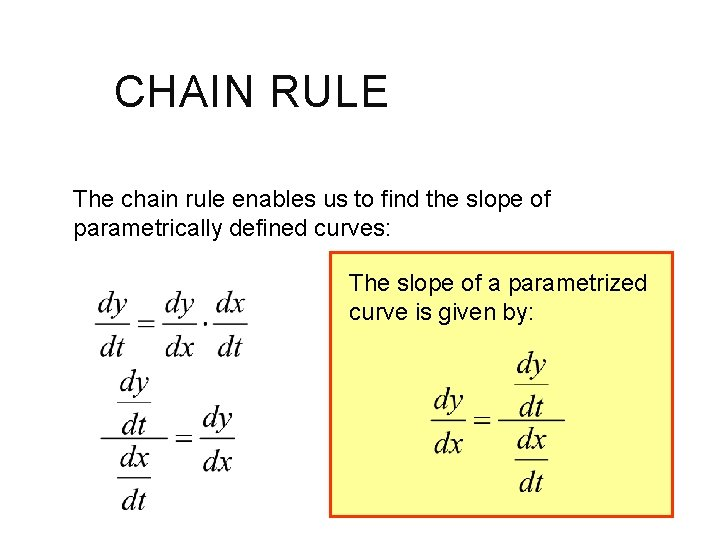CHAIN RULE The chain rule enables us to find the slope of parametrically defined curves: The slope of a parametrized curve is given by:CHAIN RULE Example: These are the equations for an ellipse.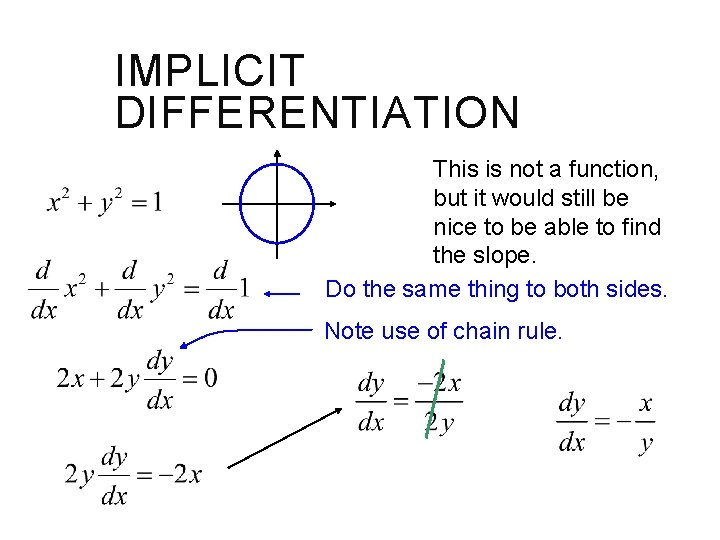IMPLICIT DIFFERENTIATION This is not a function, but it would still be nice to be able to find the slope. Do the same thing to both sides. Note use of chain rule.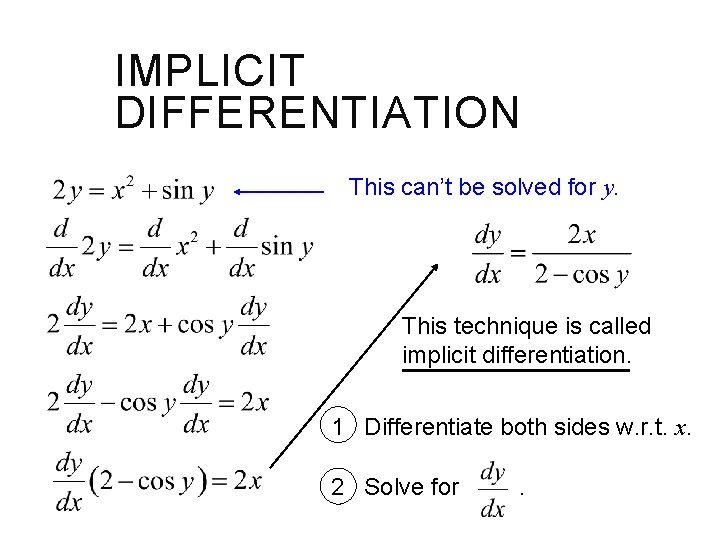IMPLICIT DIFFERENTIATION This can’t be solved for y. This technique is called implicit differentiation. 1 Differentiate both sides w. r. t. x. 2 Solve for .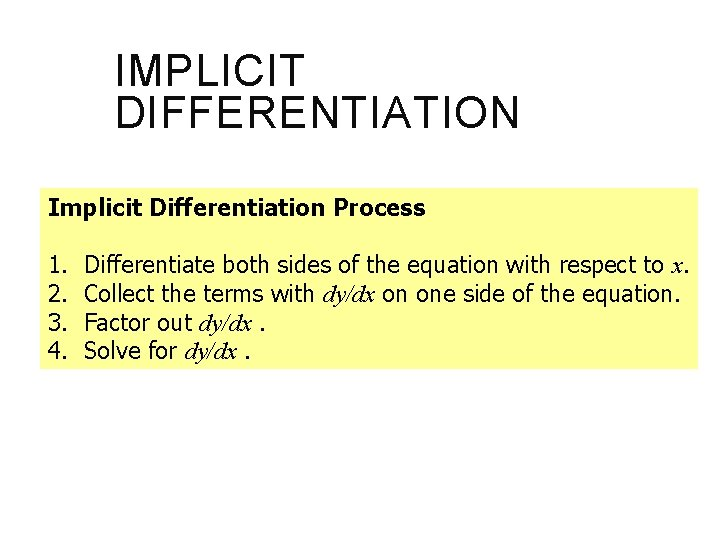IMPLICIT DIFFERENTIATION Implicit Differentiation Process 1. 2. 3. 4. Differentiate both sides of the equation with respect to x. Collect the terms with dy/dx on one side of the equation. Factor out dy/dx. Solve for dy/dx.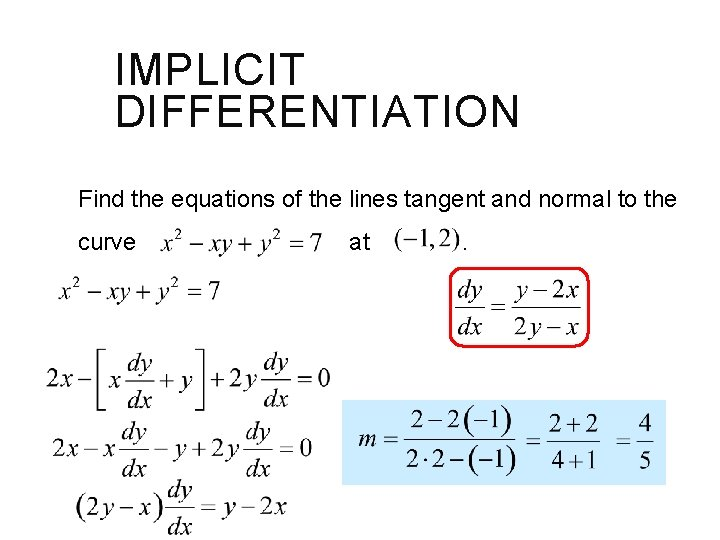IMPLICIT DIFFERENTIATION Find the equations of the lines tangent and normal to the curve at Note product rule. .IMPLICIT DIFFERENTIATION Find the equations of the lines tangent and normal to the curve at tangent: . normal:IMPLICIT DIFFERENTIATION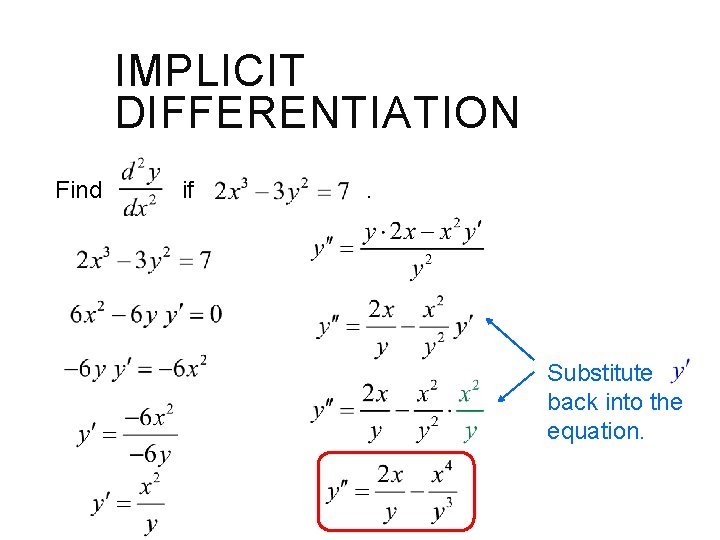IMPLICIT DIFFERENTIATION Find if . Substitute back into the equation.IMPLICIT DIFFERENTIATION Rational Powers of Differentiable Functions Power Rule for Rational Powers of x If n is any rational number, thenIMPLICIT DIFFERENTIATION Proof: Let p and q be integers with q > 0. Raise both sides to the q power Differentiate with respect to x Solve for dy/dx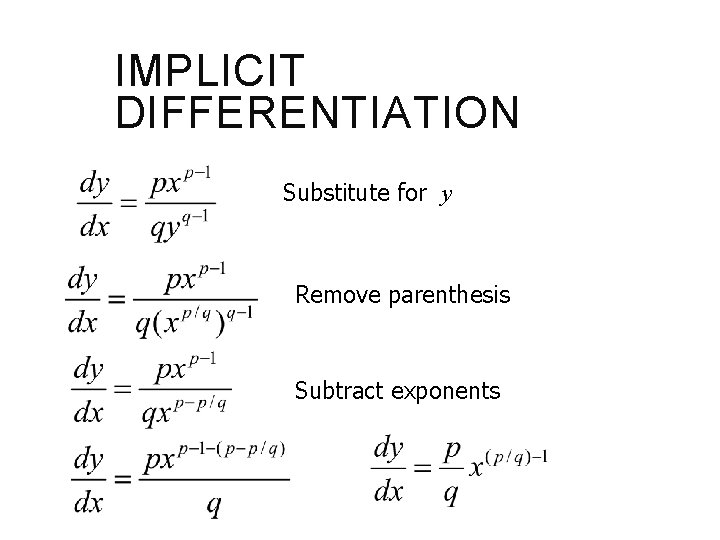IMPLICIT DIFFERENTIATION Substitute for y Remove parenthesis Subtract exponents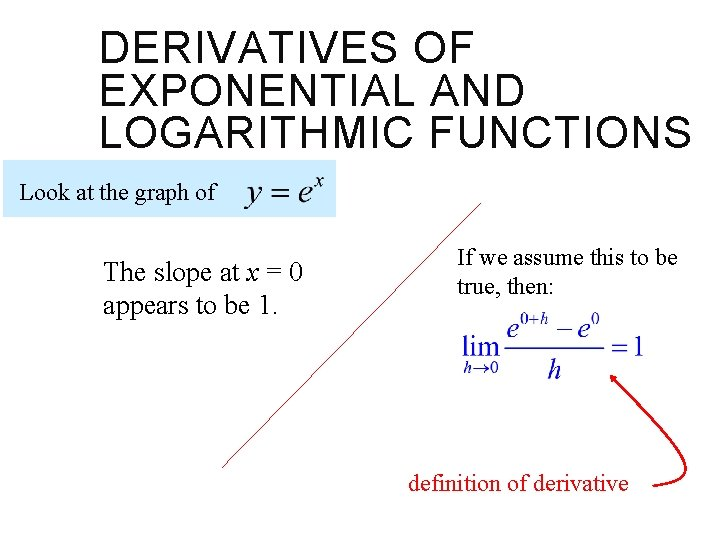DERIVATIVES OF EXPONENTIAL AND LOGARITHMIC FUNCTIONS Look at the graph of The slope at x = 0 appears to be 1. If we assume this to be true, then: definition of derivativeDERIVATIVES OF EXPONENTIAL AND LOGARITHMIC FUNCTIONS Now we attempt to find a general formula for the derivative of using the definition. This is the slope at x = 0, which we have assumed to be 1.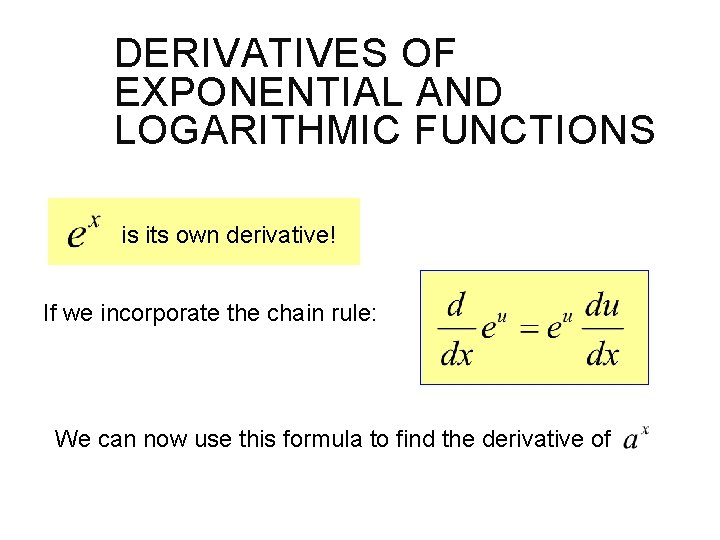DERIVATIVES OF EXPONENTIAL AND LOGARITHMIC FUNCTIONS is its own derivative! If we incorporate the chain rule: We can now use this formula to find the derivative ofDERIVATIVES OF EXPONENTIAL AND LOGARITHMIC FUNCTIONS Incorporating the chain rule:DERIVATIVES OF EXPONENTIAL AND LOGARITHMIC FUNCTIONS So far today we have: Now it is relatively easy to find the derivative of .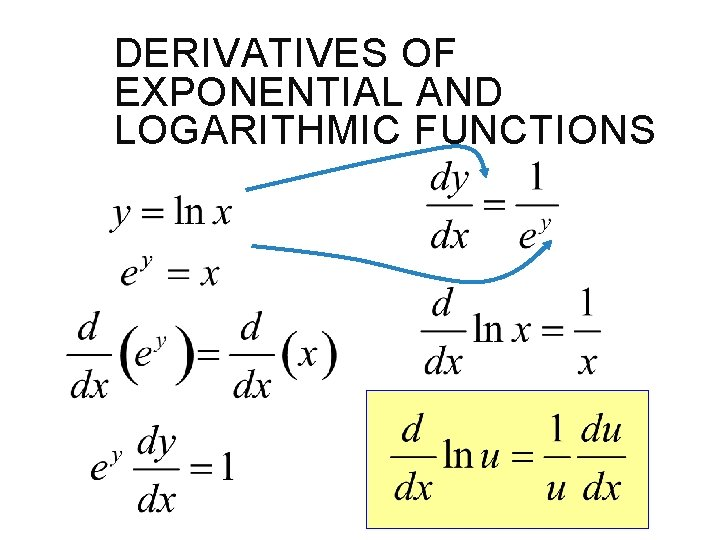DERIVATIVES OF EXPONENTIAL AND LOGARITHMIC FUNCTIONSDERIVATIVES OF EXPONENTIAL AND LOGARITHMIC FUNCTIONS To find the derivative of a common log function, you could just use the change of base rule for logs: The formula for the derivative of a log of any base other than e is: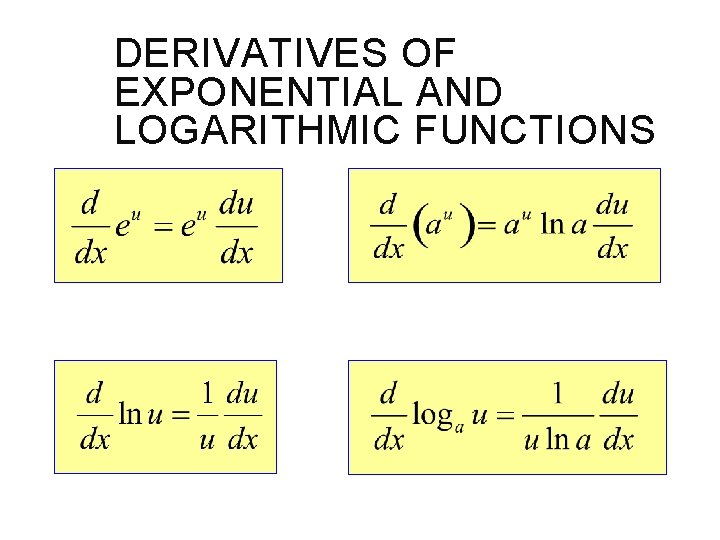DERIVATIVES OF EXPONENTIAL AND LOGARITHMIC FUNCTIONSDERIVATIVES OF EXPONENTIAL AND LOGARITHMIC FUNCTIONS Find y’DERIVATIVES OF EXPONENTIAL AND LOGARITHMIC FUNCTIONS Logarithmic differentiation Used when the variable is in the base and the exponent y = xx ln y = ln xx ln y = x ln x